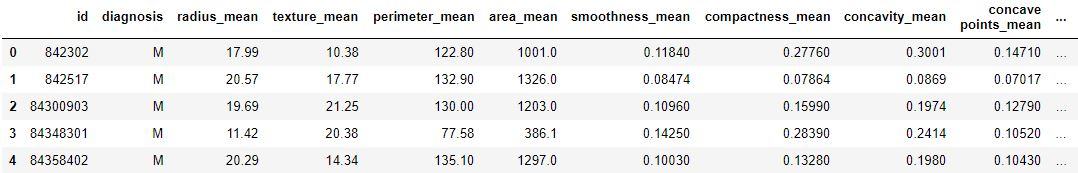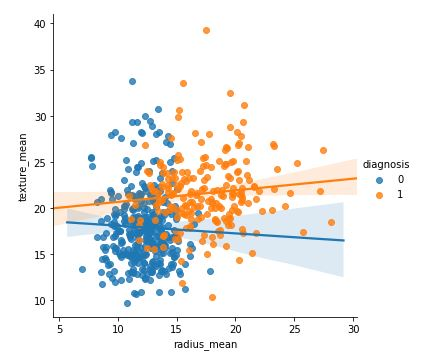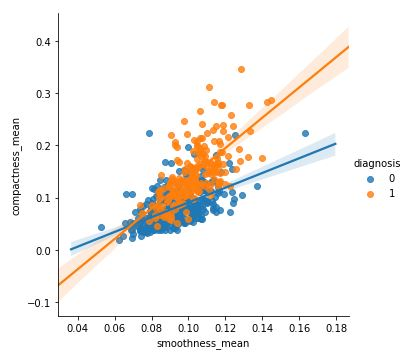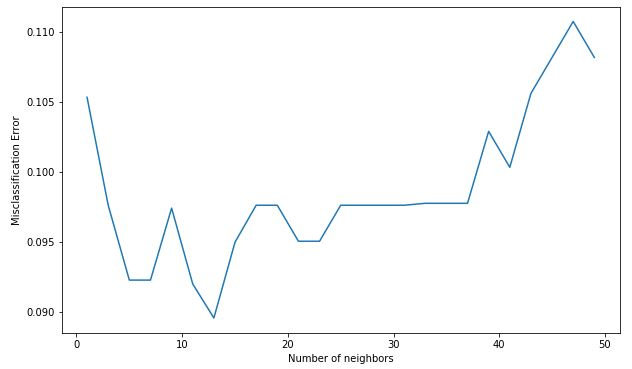GeeksforGeeks App
Open AppBrowser
Continue

# ML | Kaggle Breast Cancer Wisconsin Diagnosis using KNN and Cross Validation

Dataset :
It is given by Kaggle from UCI Machine Learning Repository, in one of its challenges.
https://www.kaggle.com/uciml/breast-cancer-wisconsin-data. It is a dataset of Breast Cancer patients with Malignant and Benign tumor.
K-nearest neighbour algorithm is used to predict whether is patient is having cancer (Malignant tumour) or not (Benign tumour).

Implementation of KNN algorithm for classification.

Code : Importing Libraries

 `# performing linear algebra``import` `numpy as np `` ` `# data processing``import` `pandas as pd`` ` `# visualisation``import` `matplotlib.pyplot as plt`

 `df ``=` `pd.read_csv(``"..\\breast-cancer-wisconsin-data\\data.csv"``)`` ` `print` `(data.head)`

Output :Code: Data Info

 `df.info()`

Output :

```RangeIndex: 569 entries, 0 to 568
Data columns (total 33 columns):
id                         569 non-null int64
diagnosis                  569 non-null object
texture_mean               569 non-null float64
perimeter_mean             569 non-null float64
area_mean                  569 non-null float64
smoothness_mean            569 non-null float64
compactness_mean           569 non-null float64
concavity_mean             569 non-null float64
concave points_mean        569 non-null float64
symmetry_mean              569 non-null float64
fractal_dimension_mean     569 non-null float64
texture_se                 569 non-null float64
perimeter_se               569 non-null float64
area_se                    569 non-null float64
smoothness_se              569 non-null float64
compactness_se             569 non-null float64
concavity_se               569 non-null float64
concave points_se          569 non-null float64
symmetry_se                569 non-null float64
fractal_dimension_se       569 non-null float64
texture_worst              569 non-null float64
perimeter_worst            569 non-null float64
area_worst                 569 non-null float64
smoothness_worst           569 non-null float64
compactness_worst          569 non-null float64
concavity_worst            569 non-null float64
concave points_worst       569 non-null float64
symmetry_worst             569 non-null float64
fractal_dimension_worst    569 non-null float64
Unnamed: 32                0 non-null float64
dtypes: float64(31), int64(1), object(1)
memory usage: 146.8+ KB
```

Code: We are dropping columns – ‘id’ and ‘Unnamed: 32’ as they have no role in prediction

 `df.drop([``'Unnamed: 32'``, ``'id'``], axis ``=` `1``)``print``(df.shape)`

Output:

`(569, 31)`

Code: Converting the diagnosis value of M and B to a numerical value where M (Malignant) = 1 and B (Benign) = 0

 `def` `diagnosis_value(diagnosis):``    ``if` `diagnosis ``=``=` `'M'``:``        ``return` `1``    ``else``:``        ``return` `0`` ` `df[``'diagnosis'``] ``=` `df[``'diagnosis'``].``apply``(diagnosis_value)`

Code :

 `sns.lmplot(x ``=` `'radius_mean'``, y ``=` `'texture_mean'``, hue ``=` `'diagnosis'``, data ``=` `df)`

Output:

``````

Code :

 `sns.lmplot(x ``=``'smoothness_mean'``, y ``=` `'compactness_mean'``, ``           ``data ``=` `df, hue ``=` `'diagnosis'``)`

Output:Code : Input and Output data

 `X ``=` `np.array(df.iloc[:, ``1``:])``y ``=` `np.array(df[``'diagnosis'``])`

Code : Splitting data to training and testing

 `from` `sklearn.model_selection ``import` `train_test_split``X_train, X_test, y_train, y_test ``=` `train_test_split(``    ``X, y, test_size ``=` `0.33``, random_state ``=` `42``)`

Code : Using Sklearn

 `knn ``=` `KNeighborsClassifier(n_neighbors ``=` `13``)``knn.fit(X_train, y_train)`

Output:

```KNeighborsClassifier(algorithm='auto', leaf_size=30,
metric='minkowski', metric_params=None,
n_jobs=None, n_neighbors=13, p=2,
weights='uniform')
```

Code : Prediction Score

 `knn.score(X_test, y_test)`

Output:

```0.9627659574468085
```

Code : Performing Cross Validation

 `neighbors ``=` `[]``cv_scores ``=` `[]`` ` `from` `sklearn.model_selection ``import` `cross_val_score``# perform 10 fold cross validation``for` `k ``in` `range``(``1``, ``51``, ``2``):``    ``neighbors.append(k)``    ``knn ``=` `KNeighborsClassifier(n_neighbors ``=` `k)``    ``scores ``=` `cross_val_score(``        ``knn, X_train, y_train, cv ``=` `10``, scoring ``=` `'accuracy'``)``    ``cv_scores.append(scores.mean())`

Code : Misclassification error versus k

 `MSE ``=` `[``1``-``x ``for` `x ``in` `cv_scores]`` ` `# determining the best k``optimal_k ``=` `neighbors[MSE.index(``min``(MSE))]``print``(``'The optimal number of neighbors is % d '` `%` `optimal_k)`` ` `# plot misclassification error versus k``plt.figure(figsize ``=` `(``10``, ``6``))``plt.plot(neighbors, MSE)``plt.xlabel(``'Number of neighbors'``)``plt.ylabel(``'Misclassification Error'``)``plt.show()`

Output:

```The optimal number of neighbors is 13
```My Personal Notes arrow_drop_up